# Proficient Key Tree Structure for Secure Dynamic Groups

2220 words (9 pages) Essay

5th Apr 2018 Computer Science Reference this

Tags:

Disclaimer: This work has been submitted by a university student. This is not an example of the work produced by our Essay Writing Service. You can view samples of our professional work here.

Any opinions, findings, conclusions or recommendations expressed in this material are those of the authors and do not necessarily reflect the views of UKEssays.com.

Secure Group Communication ensures forward secrecy and backward secrecy of the data. It is the responsibility of the Group Center (GC) to disallow new members to have access to previous data called backward secrecy and existing members who have left the group to have further access to data called forward secrecy. Forward and backward secrecy can be ensured by updating the keys whenever a join/leave request is handled. This process is called as re-keying. The tree structure is appropriate for maintaining keys. Most of the schemes use binary tree structure for maintaining keys. The Key tree structure uses three kinds of keys such as Traffic Encryption Key (TEK), Key Encryption Key (KEK) and Individual Key (IK). TEK is the top level key called Group key, KEKs are the intermediate level keys called subgroup keys and IKs are the leaf level keys.

Figure 3.1 shows the binary tree with 3-levels, which keeps keys at all levels. Based on the number of levels in the binary tree, the height of the tree (H) is calculated. Therefore the height H is 3 since there are three levels in the binary tree. Multicast group members are inserted into the tree only at leaf level. The number of nodes is 2n+1-1 and group members are 2n where ‘n’ is the number of levels in the tree.

Here, there are eight members in the multicast group and are represented by M1 to M8. The top level key K0 is the TEK, K1 to K6 are KEKs and IK1 to IK8 are the member’s private keys.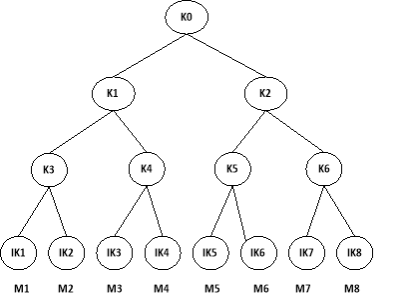Figure 3.1 3-level Binary Tree

In the key tree based group key management, the Key Centre (KC) delivers new keys to the group members by encrypting them with older keys. Then, the group members have to decrypt the encrypted keys with their old keys. All these encryption and decryption computations amplify the load on KC, resulting in delay to espouse the group key. It also increases power consumption to acquire the new group key.

All keys must be stored in the memory of communication devices and group members have to accumulate the group keys in a secure memory like Universal Subscriber Identity module (USIM) to safeguard from various kinds of attacks and intrusions. Also, each program requires memory to reserve their secure keys. Since the memory space is limited, the number of keys to be stored must also be minimised. Keeping these constraints in sight, this work suggests the proficient key tree structure, considering the efficiencies of computation and storage costs.

3.1 PROFICIENT KEY TREE STRUCTURE (PKS)

The proficient key tree structure consists of two parts in which the lower part constitutes of binary trees to minimise the communication cost and the upper part consists of flat trees up to 2 levels to alleviate the key computation and key storage costs. The TEK is directly connected to the KEKs on the top part of the tree so that it is a subset of the level homogeneous tree.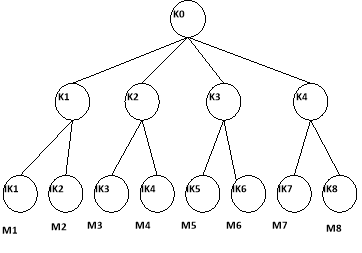Figure 3.2 Proficient Key Tree Structure with 1 flat level

Figure 3.2 shows the proficient key tree with 1flat level and 1 binary level. A Flat tree is used for reducing the computation and storage costs. From the binary key tree, one TEK, 6 KEKs and 8 IKs are stored for 8 members at three levels. Compared to the 3-level binary key tree, l-flat level PKS maintains all 8 members at 2 levels and it requires 1 TEK, 4 KEKs and 8IKs. The number of KEK is reduced if flat tree is used. This reduces key storage and key computation costs. In case of 2 flat levels and 1 binary level, 1 KEK, 16 KEKs and 32 IKs are maintained for 32 members at 3 levels.

Figure 3.3 shows the proficient key tree with 2 flat and 1 binary level. Joining and Leaving are two important operations in a multicast group.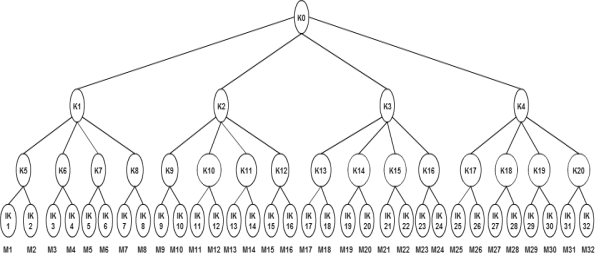Figure 3.3 Proficient Key Tree Structure with 2 flat levels and 1 binary level

If the member M24 wants to leave the group, some key updates are required for maintaining confidentiality and integrity of data. The keys K0, K3 and K16 should be updated as a part of re-keying process. The Key Centre selects a key for encryption of the new key and this new key must be kept secret from the leaving member. This ensures the property of forward secrecy.

3.1.1 Batch Re-keying

When multiple members want to leave from the multicast group, the key updates are done as a batch called batch re-keying (Li et al 2001). In batch re-keying, the interval for which key server waits, is termed as re-key interval. The entire join and leave requests are collected during this re-key interval to generate new keys. Moreover, it also constructs a re-key message and multicasts it.

Batch re-keying improves efficiency because the number of re-key messages to be signed is reduced. One message is signed for a batch of requests unlike one for each. Batch re-keying takes advantage of the possible overlap of new keys for multiple re-key requests.

3.1.2 Join Operation

When a new member (Ui) wishes to join the group, the member has to send a request. In addition to the information of existing group members, the Key Distribution Center requires the new level K’ to insert the member into the tree (Figure 3.4). If the performance of the joining member is worst, then the new level K’ should be smaller than the level of present key tree structure (K). The following steps are followed to join the member in the multicast group.

Step 1: Receive join request from the new member to the multicast group

Step 2: Determine the level of the current tree, K and new level required K’

Step 3: If K’ is greater than or equal to K, check whether the tree is complete

Step 4: If the tree is a complete tree, broadcast the remove key message that are on the same level K

Step 5: If K’ is less than K, set K as K’ and check whether the tree is complete.

Step 6: If the tree is complete, broadcast remove key messages that are on a higher level than or same level as K’ else broadcast that are on a higher level than K’.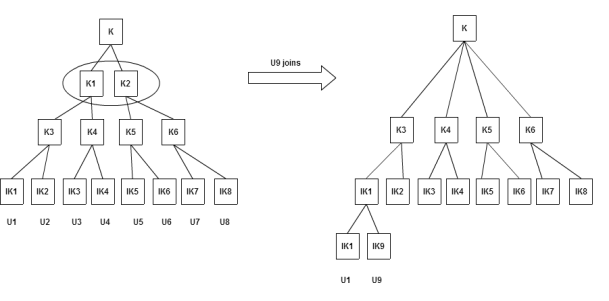Figure 3.4 Key tree updates when user joins

From the first part of the Figure 3.4, eight members are on the leaf level and the height of the lower part of the tree K is 2. If the member U9 joins into the tree, it is inserted at the leaf level. It requires one more level since the tree is complete. Therefore, the new level K’ is 3. In order to make the tree into a PKS tree, it needs to maintain the same level and the flat level is to be introduced. Therefore, the existing key tree structure is to be updated and it looks like the second part of the Figure 3.4. K1 and K2 are removed and the top level node is directly connected to K3, K4, K5 and K6 nodes after adding the new member at the leaf level.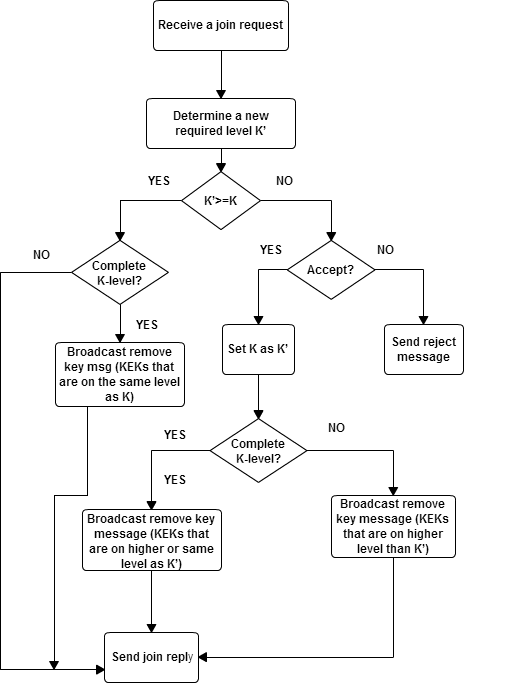Figure 3.5 Join Operation in the PKS tree

If K’ is greater than or equal to K, the KC need not to change the key tree level, excluding the case when the present key tree is a complete K-level PKS. In case of complete PKS, the key tree structure needs one more level to accommodate new members and to do so, KC should broadcast the remove key message to exterminate the KEKs in level K as in Figure 3.5.

If K’ is less than K, it indicates that the height of PKS needs to be lowered from K+1 to K’+1 in order to support the new member. As lowering the height increases the communication cost depends on KC whether to accept the new join request or not. If such addition increases the communication cost, then Key Centre can reject the request. If the tree is complete PKS, KC has to broadcast the remove key message for removing the KEKs on same or higher level than K’.

3.1.3 Leave Operation

If a group member wants to leave, it has to send a ‘Leave Request’ to the Key Centre. After receiving the request from the member(s), KC has to update the subgroup keys to maintain confidentiality and secrecy of communication. After updating the group keys, it may be a case that the height of the tree is changed due to the empty positions created as a result of leaving members. In such scenarios, height is recalculated for the tree structure’s lower part i.e. K”. In addition to this, if a group member leaves the group then the height of the tree is updated. Thus, a newly required level K’ is determined by considering the memory space and computation power of the remaining members.

The following steps are followed to leave the member into the multicast group.

Step 1: Receive a leave request from the member

Step 2: Update new KEKs

Step 3: Recalculate the height of the changed tree, K’’

Step 4: Determine a new required level K’

Step 5: If both K’’ and K’ are not equal and heightening the level of the tree, create levels from K’’+1 to K’.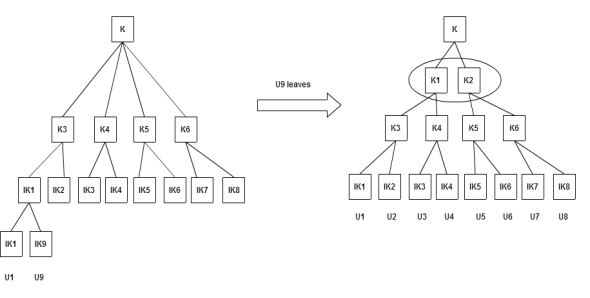Figure 3.6 Key tree updates when a user leaves

From the Figure 3.6, the member U9 wants to leave the multicast group. K is 2 and the height of the lower part of the changed key tree K” is 1. If K” is smaller than K, the KC decides to heighten the height of the tree. If it so, it generates new KEKs on level two.

If K” is equal to K’ then the height of the changed key tree is same as the height of key tree which is required for communication. In this scenario, no changes will be done in the height of the key tree structure. If K” < K’, Key Centre determines whether the height of the tree needs to be raised or not. In leave operation, if the height of flat tree gets increased more than 2 levels, KC broadcasts the “Remove Key” message and removes a key level in such a way that the flat levels do not exceed 2 as shown in Figure 3.7.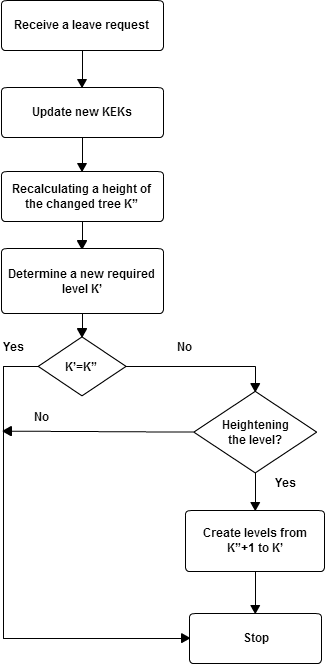Figure 3.7 Leave operation in the PKS tree

3.2 PERFORMANCE METRICS

The performance of the PKS tree structure is evaluated in different aspects of costs such as Key computation, Key storage.

3.2.1 Key Computation Cost

In a key tree structure, three keys are considered. They are group key (TEK), subgroup key (KEK) and Individual Keys (IK). As the level in a key tree structure increases, the number of keys for the group or member also increases. The depth of a binary tree equals to the integer part of log2n, where‘n’denotes the number of nodes on the balanced tree. The PKS tree maintains two types of levels. They are the flat levels (fl) and binary levels (bl). The height (H) of the tree is the number of flat and binary levels, i.e., H=fl+bl. Each member has one key on each level. Therefore, the number of key computations is same as the height of the key tree.

3.2.2 Key Storage Cost

Key storage cost is defined as the number of keys stored by each member in the multicast group and KC. Each member has to store one key on each level. Therefore, it is the height of the tree ‘fl+bl’ for the PKS tree and ‘bl’ for the binary tree.

3.3 SUMMARY

Proficient tree based re-keying algorithm is proposed so that it reduces the number of re-keying operations per join/leave request. The Proficient Key Tree Structure has two parts in which the lower part constitutes of binary trees to minimise the communication cost and the upper part consists of flat trees to alleviate the key computation and key storage costs.

The time efficiency of all key tree structures is based on the height of the trees. The height is the number of binary levels of the binary tree and the height of the PKS tree is based on the number of flat and binary levels. It is concluded that the time efficiency of the PKS tree is less than the binary tree since the height of PKS is lesser than the binary key tree for the specified number of members in the multicast group.

Thus, the proficient key tree structure for re-keying is proposed in this chapter. It gives the complete picture about joining and leaving of users in the multicast group and the key computations during re-keying operations. Multilevel encryption and decryption using graceful codes are discussed in the following chapter.

## Related Services

View all

### DMCA / Removal Request

If you are the original writer of this essay and no longer wish to have your work published on the UKDiss.com website then please:

Related Services